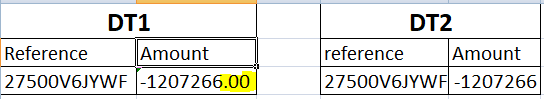# Unable to get matched records with LINQ

Hi,
I am trying to get matched records from two DT’s but unfortunately unable to do so, whereas when checking manually I find few records matching, but when using LINQ to get the same, I failed to do so, help me rectify-

Note - the amount field having negative values in both the DT.

``````(From a In DT1.AsEnumerable()
Where DT2.AsEnumerable.Any(Function (b) a("Reference").ToString.Trim.Equals(b("reference").ToString.Trim) And a("Amount").ToString.Equals(b("Amount").ToString))
Select a).CopyToDataTable()
``````Try this linq query

dt_CommonRows = dt1.AsEnumerable().Intersect(dt2.AsEnumerable(),System.Data.DataRowComparer.Default).CopyToDataTable

Mark it as solution if it resolves ur query

Regards

Nived NHappy AutomationI have to be field specific while comparing, & I can’t compare with whole table as there are chances of duplicate in DT1

I am not much sure whether it works

Then try like this

(From a In DT1.AsEnumerable()
From b in DT2.AsEnumerable()
Where a(“Reference”).ToString.Trim.Equals(b(“reference”).ToString.Trim) And a(“Amount”).ToString.Equals(b(“Amount”).ToString)
Select a).CopyToDataTable()

Try this

1 Like

Is there any chance decimal/double value could be the problem?I think yes

Because there is .00 which distinguishes both

So better convert to int format then check

Tried this below code and it worked for me.

``````(From a In DT1.AsEnumerable()
Where DT2.AsEnumerable.Any(Function (b) a("Reference").ToString.Trim.Equals(b("reference").ToString.Trim) And Convert.ToDouble(a("Amount")).Equals(Convert.ToDouble(b("Amount"))))
Select a).CopyToDataTable()
``````

Did the code

Work for you @indrajit.shah

no, but if we could convert the amount to double in the same code, it sure will work1 Like

@indrajit.shah
Perfect. My recomendation would look as the same for the first step

1 Like

can you help me with how to achieve the below scenario?

str = 08022021 ---- its date in ddMMyyyy format
I want to change it to 08-02-2021

other than datetime.ParseExact, can we add - after dd and mm using something like position based to replace?

we prefer to do it on datetime parsexact as we can do the bot more reliable. Unforseen values let fail the parsing and can be detected.
Any constraints why it should be done other than datetime.ParseExact?

doing it with string base can maybe use strategies like

2 Likes

This topic was automatically closed 3 days after the last reply. New replies are no longer allowed.